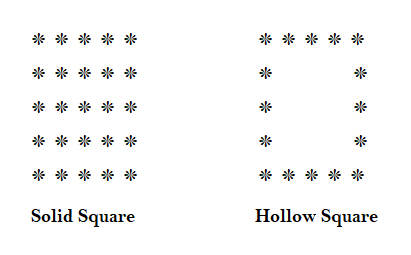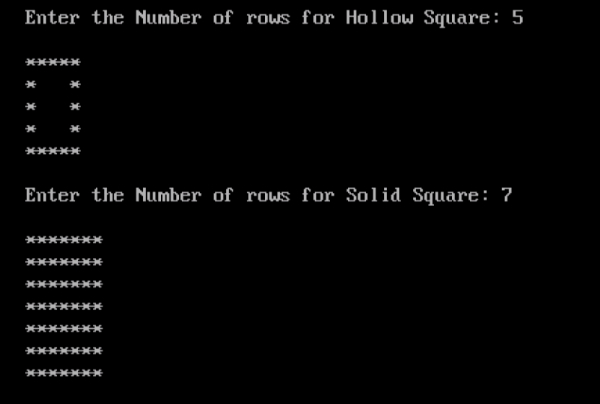# Program to print solid and hollow square patterns in C

## Program Description

In geometry, a square is a regular quadrilateral, which means that it has four equal sides and four equal angles.

Solid and Hollow Square will appear as shown below## Algorithm

For Solid Square −

Accept the Number of Rows from the user to draw the Solid Square
For each Row, Print * for each Column to draw the Solid Square

For Hollow Square −

Accept the Number of Rows from the user to draw the Hollow Square
For the First and Last Row, Print * for each Column
For the Remaining Rows, Print * for the first and Last Column.

## Example

/* Program to print hollow and Solid Square pattern */
#include <stdio.h>
int main(){
int r, c, rows; //Hollow Rhombus
int r1,c1, rows1; //Solid Rhombus
clrscr();
/* Hollow Square */
printf("Enter the Number of rows for Hollow Square: ");
scanf("%d", &rows);
printf("");
for (r=1; r<=rows; r++){
if (r==1 || r==rows){
for (c=1; c<=rows; c++){
printf("*");
}
}
else{
for (c=1; c<=rows; c++){
if (c==1 || c==rows){
printf("*");
}
else{
printf(" ");
}
}
}
printf("");
}
printf("");
/* Solid Square */
printf("Enter the Number of rows for Solid Square: ");
scanf("%d", &rows1);
printf("");
for (r1=1; r1<=rows1; r1++){
for (c1=1; c1<=rows1; c1++){
printf("*");
}
printf("");
}
getch();
return 0;
}

## Output